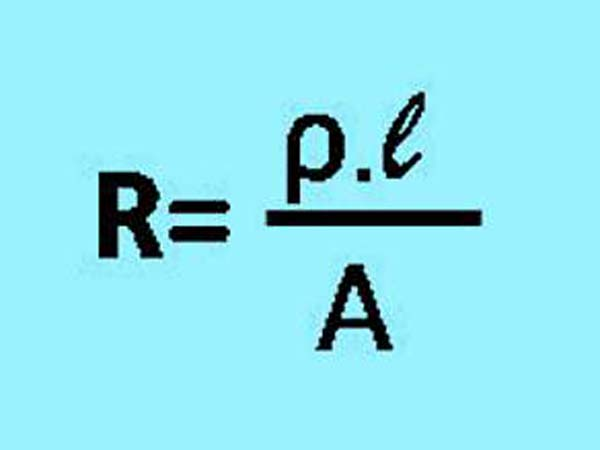# Calculation of Conductor Resistance

Resistance is influenced by the length and Conductor Resistivity, In an electrical installation, requires various parts in it, both the main part and the parts as a complement to the electrical installation, But in general, in every electrical installation there are three main parts.

Three main parts to the electrical installation, namely:

1. Power Source
Power source or also called electricity generation is a device that can produce electrical energy, Some examples of power sources include: Generators, Solar Cell, Battery and others.

2. Conductor
Conductor is any material that has the property to conduct electricity properly, this conductor serves to conduct electricity from an electric source to the electrical load, Conductors commonly used include: Electric cables made of copper or aluminum.

Electric load is everything that works by using electrical energy as a power source, Such as: Lights, Fans, Electromotor, and others.

In the science of electricity, we know the term Ohm's Law, which reads:
"The magnitude of the Electric Current flowing through an electric conductor will be directly proportional to the value of the Voltage and inversely proportional to the Resistance (Ohm)".
Conductor is Very important Part in electrical installation, therefore we need a Conductor material that has a resistivity as small as possible to be used as a conductor of electricity, Because the smaller of the conductor resistiviy, the smaller electrical losses that occur in an electrical installation.

Calculation of how big resistance is generated from the conductor in an electrical installation, then the following formula is used,

## How to Calculate of Conductor Resistance

The Formula for Calculating Conductor ResistanceCalculation of Conductor resistance
• R = Resistance in units of Ohms (Ω)
• ρ (rho) = Conductor Resistivity in units of Ohm meter (Ω mm² / m)
• l = length of conductor in meters (m)
• A = Area of conductor cross section in units of meters squared (mm²)
From the formula above, we can conclude that:

Conclusion:
• The longer the distance of an installed electrical conductor, the greater the resistance of the conductor.
• The greater the value of Conductor resistance (ρ), the greater the value of resistance.
• The greater the cross-sectional area of a conductor, the smaller the value of the conductor resistance.

Therefore, to produce the smallest resistance value, a conductor with a large cross-sectional area is needed. Or by choosing Conductor materials that has a small resistivity (ρ), Electrical conductors are generally used in an electrical installation using conductors made from copper, this is because the Copper material has a resistivity (ρ) that is small enough and good for conducting electricity.

Here are some types of conductors which are often used as conductors of electricity and the values of resistivity (ρ):
• Aluminum has resistivity (ρ) 0.0000000265 Ohm-meters.
• Copper has resistivity (ρ) 0.0000000172 Ohm-meters.
• Silver has resistivity (ρ) 0.0000000159 Ohm-meters.
• Iron has resistivity (ρ) 0.0000000971 Ohm-meters.
Resistivity (Rho) is:
Value of resistance in Ohms units, which produced by conductor which has a length 1 meter and diameter 1 mm²., Resistivity Units is Ohm-Meters (Ω mm² / m).

In addition, Conductor Resistance can also be influenced by the temperature at the time of measurement.

My Electrical Diary